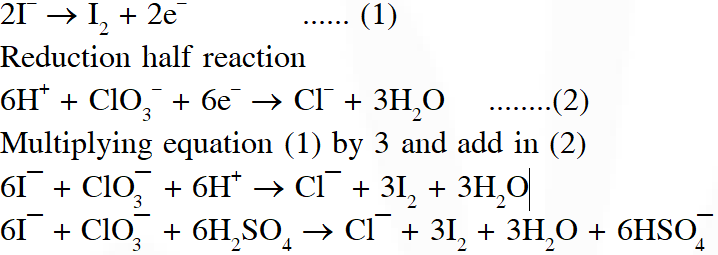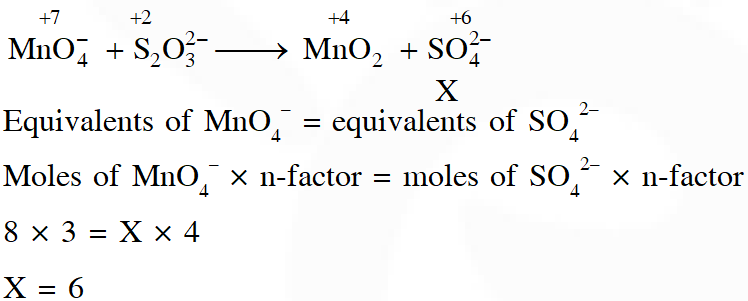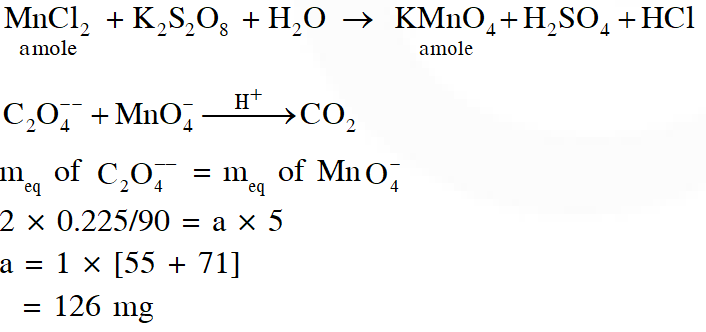# Redox Reaction - JEE Advanced Previous Year Questions with Solutions

JEE Advanced Previous Year Questions of Chemistry with Solutions are available at eSaral. Practicing JEE Advanced Previous Year Papers Questions of Chemistry will help the JEE aspirants in realizing the question pattern as well as help in analyzing weak & strong areas.   Simulator   Previous Years JEE Advance Questions
Q. Reduction of the metal centre in aqueous permanganate ion involves - (A) 3 electrons in neutral medium (B) 5 electrons in neutral medium (C) 3 electrons in alkaline medium (D) 5 electrons in acidic medium [JEE-2011]
Ans. (A,C,D)
Q. Reaction of $\mathrm{Br}_{2}$ with $\mathrm{Na}_{2} \mathrm{CO}_{3}$ in aqueous solution gives sodium bromide and sodium bromate with evolution of $\mathrm{CO}_{2}$ gas. The number of sodium bromide molecules involved in the balanced chemical equation is. [JEE- 2011]
Ans. 5
Q. Which ordering of compounds is according to the decreasing order of the oxidation state of nitrogen- (A) $\mathrm{HNO}_{3}, \mathrm{NO}, \mathrm{NH}_{4} \mathrm{Cl}, \mathrm{N}_{2}$ (B) $\mathrm{HNO}_{3}, \mathrm{NO}, \mathrm{N}_{2}, \mathrm{NH}_{4} \mathrm{Cl}$ (C) $\mathrm{HNO}_{3}, \mathrm{NH}_{4} \mathrm{Cl}, \mathrm{NO}, \mathrm{N}_{2}$ (D) $\mathrm{NO}, \mathrm{HNO}_{3}, \mathrm{NH}_{4} \mathrm{Cl}, \mathrm{N}_{2}$ [JEE- 2012]
Ans. (B)
Q. 25 mL of household bleach solution was mixed with 30 mL of 0.50 M KI and 10 mL of 4 N acetic acid. In the titration of the liberated iodine, 48 mL of 0.25 N $\mathrm{Na}_{2} \mathrm{S}_{2} \mathrm{O}_{3}$ was used to reach the end point. The molarity of the household bleach solution is (A) 0.48 M (B) 0.96 M (C) 0.24 M (D) 0.024 M [JEE- 2012]
Ans. (C) Eq. of $\mathrm{CaOCl}_{2}=\mathrm{Eq}$, of $\mathrm{Na}_{2} \mathrm{S}_{2} \mathrm{O}_{3}$ 25 × M × 2 = 48 × 0.25 $\left[2 \mathrm{H}^{+}+\mathrm{CaOCl}_{2}+2 \mathrm{e}^{-} \longrightarrow \mathrm{Ca}^{2+}+\mathrm{H}_{2} \mathrm{O}+2 \mathrm{Cl}^{-}\right]$ (nf = 2) M = 0.24
Q. For the reaction $\mathrm{I}^{-}+\mathrm{ClO}_{3}^{-}+\mathrm{H}_{2} \mathrm{SO}_{4} \rightarrow \mathrm{Cl}^{-}+\mathrm{HSO}_{4}^{-}+\mathrm{I}_{2}$ The correct statement(s) in the balanced equation is / are : (A) Stoichiometric coefficient of $\mathrm{HSO}_{4}^{-}$ is 6 (B) Iodide is oxidized (C) Sulphur is reduced (D) $\mathrm{H}_{2} \mathrm{O}$ is one of the products [JEE- 2014]
Ans. (A,B,D) Oxidation half reaction :Q. In neutral or faintly alkaline solution, 8 moles permanganate anion quantitatively oxidize thiosulphate anions to produce X moles of a sulphur containing product. the magnitude of X is [JEE - Adv. 2016]
Ans. 6Q. To measure the quantity of $\mathrm{MnCl}_{2}$ dissolved in an aqueous solution, it was completely converted to $\mathrm{KMnO}_{4}$ using the reaction, $\mathrm{MnCl}_{2}+\mathrm{K}_{2} \mathrm{S}_{2} \mathrm{O}_{8}+\mathrm{H}_{2} \mathrm{O} \rightarrow \mathrm{KMnO}_{4}+\mathrm{H}_{2} \mathrm{SO}_{4}+$ HCl (equation not balanced). Few drops of concentrated HCl were added to this solution and gently warmed. Further, oxalic acid (225 g) was added in portions till the colour of the permanganate ion disappeard. The quantity of MnCl2 (in mg) present in the initial solution is (Atomic weights in $\mathrm{g}$ mol $^{-1}: \mathrm{Mn}=55, \mathrm{Cl}=35.5$ ) [JEE - Adv. 2018]
Ans. 126